# NCERT Solutions for Class 10 Maths Exercise 7.1## myCBSEguide App

Complete Guide for CBSE Students

NCERT Solutions, NCERT Exemplars, Revison Notes, Free Videos, CBSE Papers, MCQ Tests & more.

NCERT solutions for Maths Coordinate Geometry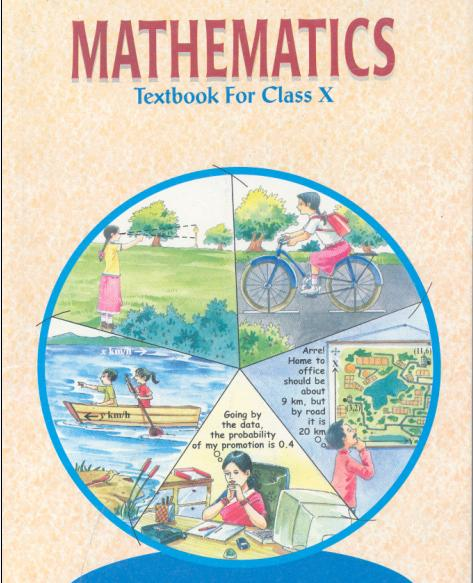## NCERT Solutions for Class 10 Maths Coordinate Geometry

###### 1. Find the distance between the following pairs of points:

(i) (2, 3), (4,1)

(ii) (–5, 7), (–1, 3)

(iii) (a, b), (–a, –b)

Ans. (i) Applying Distance Formula to find distance between points (2, 3) and (4,1), we get

d =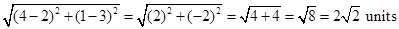(ii) Applying Distance Formula to find distance between points (–5, 7) and (–1, 3), we get

d =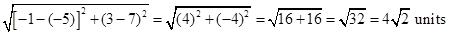(iii) Applying Distance Formula to find distance between points (a, b) and (–a, –b), we get

d =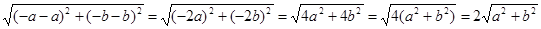NCERT Solutions for Class 10 Maths Exercise 7.1

###### 2. Find the distance between the points (0, 0) and (36, 15). Also, find the distance between towns A and B if town B is located at 36 km east and15 km north of town A.

Ans. Applying Distance Formula to find distance between points (0, 0) and (36, 15), we get

d =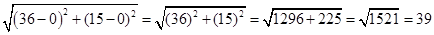Town B is located at 36 km east and15 km north of town A. So, the location of town A and B can be shown as: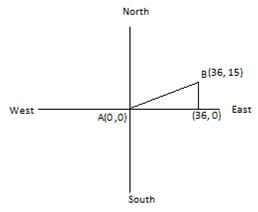Clearly, the coordinates of point A are (0, 0) and coordinates of point B are (36, 15).

To find the distance between them, we use Distance formula:

d =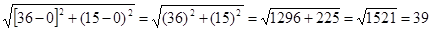km

NCERT Solutions for Class 10 Maths Exercise 7.1

###### 3. Determine if the points (1, 5), (2, 3) and (–2, –11) are collinear.

Ans. Let A = (1, 5), B = (2, 3) and C = (–2, –11)

Using Distance Formula to find distance AB, BC and CA.

AB =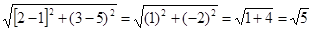BC =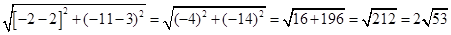CA =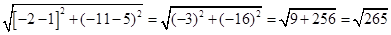Since AB + AC ≠ BC, BC + AC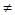AB and ACBC.

Therefore, the points A, B and C are not collinear.

NCERT Solutions for Class 10 Maths Exercise 7.1

###### 4. Check whether (5, –2), (6, 4) and (7, –2) are the vertices of an isosceles triangle.

Ans. Let A = (5, –2), B = (6, 4) and C = (7, –2)

Using Distance Formula to find distances AB, BC and CA.

AB =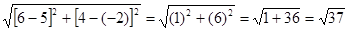BC =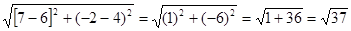CA =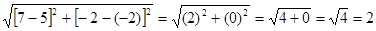Since AB = BC.

Therefore, A, B and C are vertices of an isosceles triangle.

NCERT Solutions for Class 10 Maths Exercise 7.1

###### 5. In a classroom, 4 friends are seated at the points A (3, 4), B (6, 7), C (9, 4) and D (6, 1). Champa and Chameli walk into the class and after observing for a few minutes Champa asks Chameli. “Don’t you think ABCD is a square?”Chameli disagrees. Using distance formula, find which of them is correct.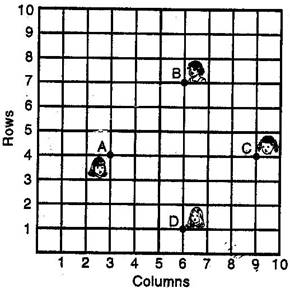Ans. We have A = (3, 4), B = (6, 7), C = (9, 4) and D = (6, 1)

Using Distance Formula to find distances AB, BC, CD and DA, we get

AB =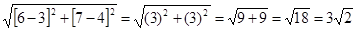BC =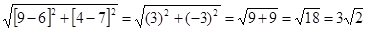CD =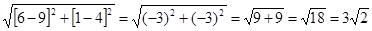DA =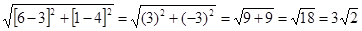Therefore, All the sides of ABCD are equal here. … (1)

Now, we will check the length of its diagonals.

AC =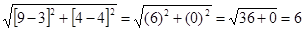BD =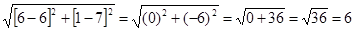So, Diagonals of ABCD are also equal. … (2)

From (1) and (2), we can definitely say that ABCD is a square.

Therefore, Champa is correct.

NCERT Solutions for Class 10 Maths Exercise 7.1

###### 6. Name the type of quadrilateral formed, if any, by the following points, and give reasons for your answer.

(i) (–1, –2), (1, 0), (–1, 2), (–3, 0)

(ii) (–3, 5), (3, 1), (0, 3), (–1, –4)

(iii) (4, 5), (7, 6), (4, 3), (1, 2)

Ans. (i) Let A = (–1, –2), B = (1, 0), C= (–1, 2) and D = (–3, 0)

Using Distance Formula to find distances AB, BC, CD and DA, we get

AB =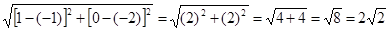BC =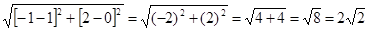CD =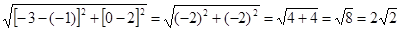DA =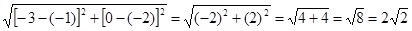Therefore, all four sides of quadrilateral are equal. … (1)

Now, we will check the length of diagonals.

AC =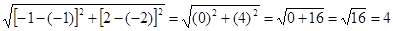BD =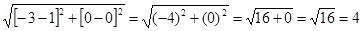Therefore, diagonals of quadrilateral ABCD are also equal. … (2)

From (1) and (2), we can say that ABCD is a square.

(ii) Let A = (–3, 5), B= (3, 1), C= (0, 3) and D= (–1, –4)

Using Distance Formula to find distances AB, BC, CD and DA, we get

AB =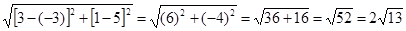BC =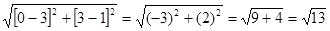CD =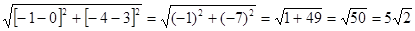DA =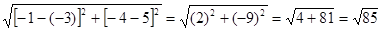We cannot find any relation between the lengths of different sides.

Therefore, we cannot give any name to the quadrilateral ABCD.

(iii) Let A = (4, 5), B= (7, 6), C= (4, 3) and D= (1, 2)

Using Distance Formula to find distances AB, BC, CD and DA, we get

AB =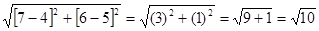BC =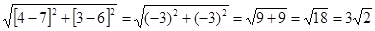CD =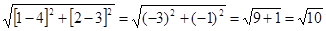DA =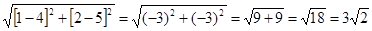Here opposite sides of quadrilateral ABCD are equal. … (1)

We can now find out the lengths of diagonals.

AC =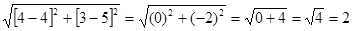BD =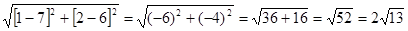Here diagonals of ABCD are not equal. … (2)

From (1) and (2), we can say that ABCD is not a rectangle therefore it is a parallelogram.

NCERT Solutions for Class 10 Maths Exercise 7.1

###### 7. Find the point on the x–axis which is equidistant from (2, –5) and (–2, 9).

Ans. Let the point be (x, 0) on x–axis which is equidistant from (2, –5) and (–2, 9).

Using Distance Formula and according to given conditions we have: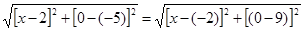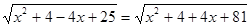Squaring both sides, we get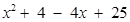=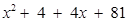⇒ −4x + 29 = 4x + 85

⇒ 8x = −56

⇒ x = −7

Therefore, point on the x–axis which is equidistant from (2, –5) and (–2, 9) is (–7, 0)

NCERT Solutions for Class 10 Maths Exercise 7.1

###### 8. Find the values of y for which the distance between the points P (2, –3) and Q (10, y) is 10 units.

Ans. Using Distance formula, we have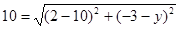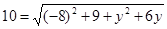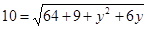Squaring both sides, we get

100 =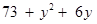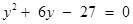Solving this Quadratic equation by factorization, we can write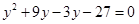⇒ y (y + 9) – 3 (y + 9) = 0

⇒ (y + 9) (y − 3) = 0

⇒ y = 3, −9

NCERT Solutions for Class 10 Maths Exercise 7.1

###### 9. If, Q (0, 1) is equidistant from P (5, –3) and R (x, 6), find the values of x. Also, find the distances QR and PR.

Ans. It is given that Q is equidistant from P and R. Using Distance Formula, we get

PQ = RQ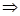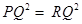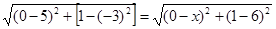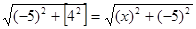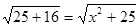Squaring both sides, we get

⇒ 25 + 16 =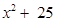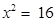⇒ x = 4, −4

Thus, Q is (4, 6) or (–4, 6).

Using Distance Formula to find QR, we get

Using value of x = 4 QR =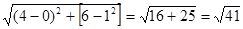Using value of x = –4 QR =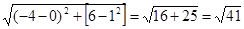Therefore, QR =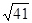Using Distance Formula to find PR, we get

Using value of x = 4 PR =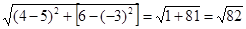Using value of x =–4 PR =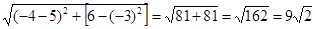Therefore, x = 4, –4

QR =, PR =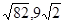NCERT Solutions for Class 10 Maths Exercise 7.1

###### 10. Find a relation between x and y such that the point (x, y) is equidistant from the point (3, 6) and (–3, 4).

Ans. It is given that (x, y) is equidistant from (3, 6) and (–3, 4).

Using Distance formula, we can write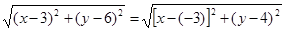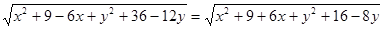Squaring both sides, we get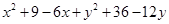=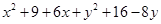⇒ −6x − 12y + 45

= 6x − 8y + 25

⇒ 12x + 4y = 20

⇒ 3x + y = 5

## NCERT Solutions for Class 10 Maths Exercise 7.1

NCERT Solutions Class 10 Maths PDF (Download) Free from myCBSEguide app and myCBSEguide website. Ncert solution class 10 Maths includes text book solutions from Mathematics Book. NCERT Solutions for CBSE Class 10 Maths have total 15 chapters. 10 Maths NCERT Solutions in PDF for free Download on our website. Ncert Maths class 10 solutions PDF and Maths ncert class 10 PDF solutions with latest modifications and as per the latest CBSE syllabus are only available in myCBSEguide.

## CBSE app for Class 10

To download NCERT Solutions for Class 10 Maths, Computer Science, Home Science,Hindi ,English, Social Science do check myCBSEguide app or website. myCBSEguide provides sample papers with solution, test papers for chapter-wise practice, NCERT solutions, NCERT Exemplar solutions, quick revision notes for ready reference, CBSE guess papers and CBSE important question papers. Sample Paper all are made available through the best app for CBSE## Test Generator

Create Papers with your Name & Logo

### 23 thoughts on “NCERT Solutions for Class 10 Maths Exercise 7.1”

1. I am setisfy by this solution

2. I want form a equilateral triangle A(a,0),B (-a ,0) C(0,a?3)

3. This educational site it is very best for students and teachers.

4. Nice

5. Thankyou

6. nice

7. Thanx?

8. thank you

11. It’s nice

12. This is very good site for students

13. This is very good site for students

14. Thankuuuuu?

15. Thank u soo much

16. Thank uhhh so muchh this helped me a lot…!! ?

17. Nice

18. Correct the steps of last solution answer is c?reject